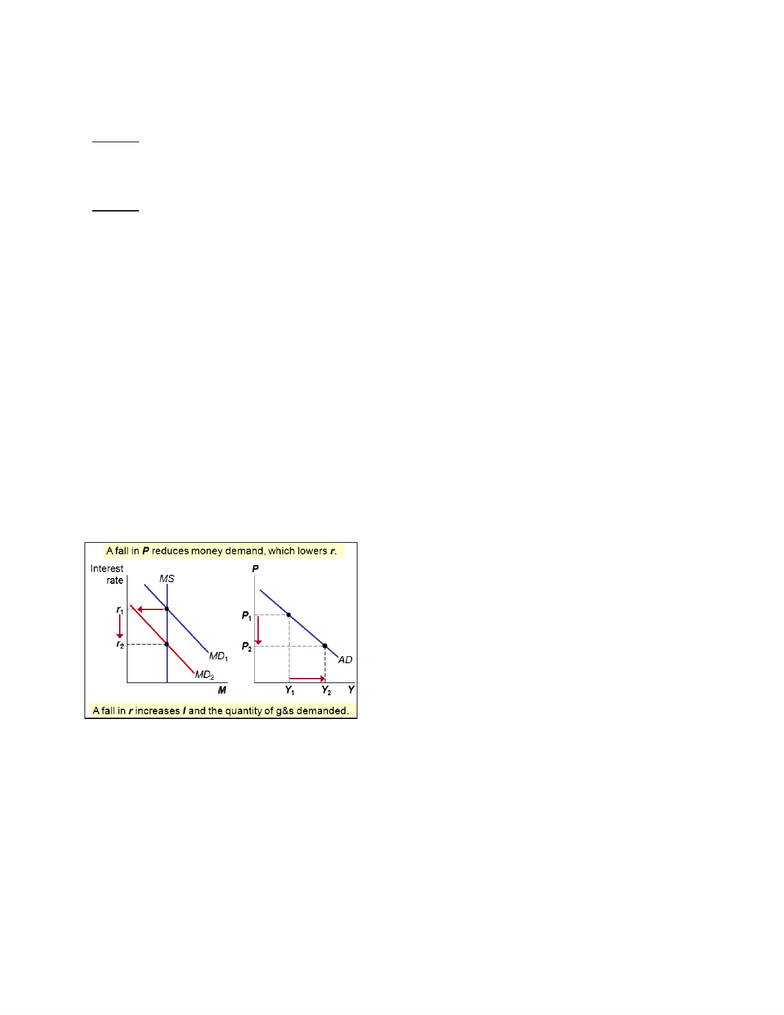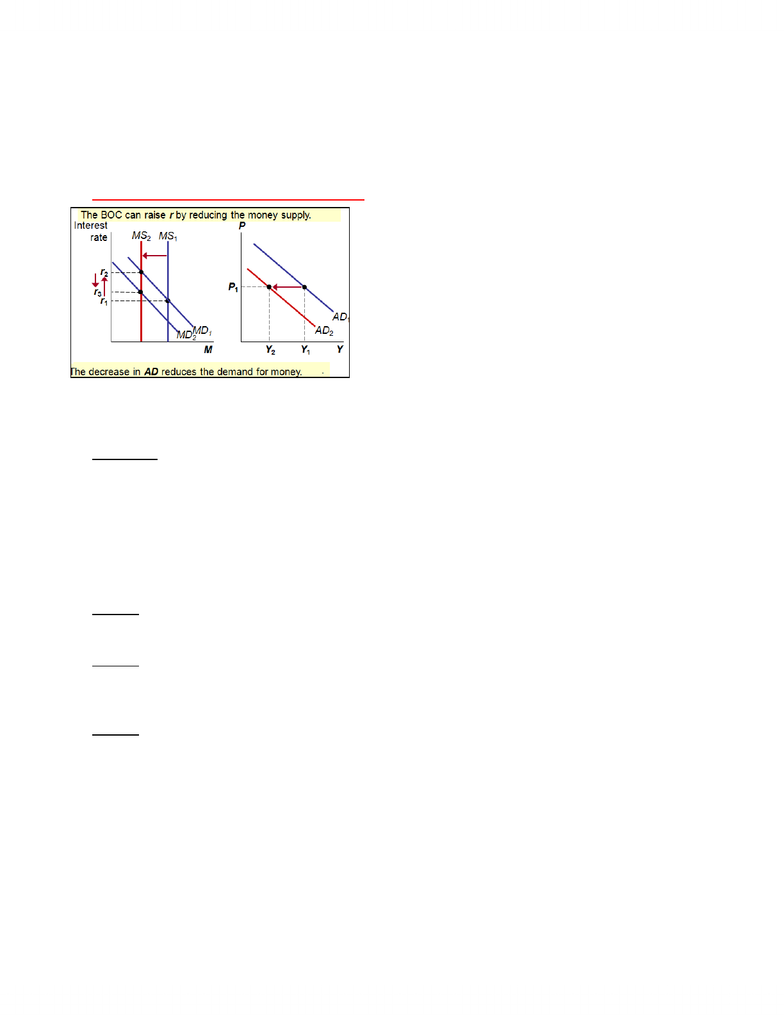# ECN 204 Lecture Notes - Open Market Operation, Fiscal Policy, Aggregate Demand

42 views8 pages
School
Department
Course
ProfessorChapter 15: Influence of Monetary and Fiscal Policy on Aggregate Demand
-Monetary and fiscal policy can each influence aggregate demand- a change in one of these policies can
lead to a short-run fluctuation in output and prices.
Money Market: Money Supply and Money Demand;
Vertical Axis- Interest Rate Horizontal Axis- Quantity of money
Aggregate Demand Curve: Vertical axis- Price Level Horizontal Axis- Quantity of Output
How Monetary Policy Influences Aggregate Demand
-The aggregate demand curve shows the total quantity of goods and services demanded in the economy
for any price level. AD slopes downward because of the wealth effect, the interest rate effect, and the
exchange-rate effect. We will focus on the interest-rate effect because it the most important in a
CLOSED economy. We will see how policy can help short run economic fluctuations.
The Theory of Liquidity Preference
Theory of Liquidity Preference: Keynes’s theory that the interest rate adjusts to bring more money
supply and money demand into balance
-A simple theory of the interest rate (denoted r), r adjusts to balance supply and demand for money
1: Money Supply.
Horizontal Axis: quantity of money, Vertical Axis: interest rate. We assume the BOC controls the money
supply directly and assumed the supply of money is not affected at all by changes in interest rate. For
this reason, the money-supply curve is a vertical line.
-The Bank of Canada alters the money of supply using two methods: the first is to change the quantity of
reserves in the banking system through what is known as an open-market operation such as BOC buying
and selling government bonds in bond market OR buying and selling foreign currencies in the market for
foreign currency exchange. The second way BOC can alter the money supply is by changing the bank
rate. The bank rate is the interest rate on the loans that the BOC makes to commercial banks.
2: Money Demand
-Money demand reflects how much wealth people want to hold in liquid form. One factor that is
important in determining the quantity of money demanded is the interest rate because it is the
opportunity cost of holding money. An increase in the interest rate raises the cost of holding money =
reducing the quantity of money demanded. A decrease in interest rate reduces the cost of holding
money and raises the quantity demanded. Therefore, the money demand curve slopes DOWNWARDS.
-Variables that influence money demand: Y, r, and P. Suppose real income (Y) rises. If Y rises, households
want to buy more goods and services, so they need more money. To get this money, they attempt to sell
some of their bonds = an increase in Y causes an increase in money demand
-People hold money in order to buy g&s. IF the dollar value of transactions increases because of an
increase in either prices or GDP, then for any given interest rate people will hold more of their assets as
money = money demand shifts to the RIGHT. If the dollar value of transaction decreases because of a
decrease in price or GDP, then for any given interest rate, people will hold less of their assets as money
= money demand shifts to the LEFT.
Unlock document

This preview shows pages 1-3 of the document.
Unlock all 8 pages and 3 million more documents.Exercise 1: The determinants of money demand
A: Suppose r rises, but Y and P are unchanged. What happens to money demand?
Answer: r is the opportunity cost of holding money. An increase in r reduces money demand:
households attempt to buy bonds to take advantage of the higher interest rate. Hence, an increase in r
causes a decrease in money demand, other things equal.
B: Suppose P rises, but Y are r are unchanged. What happens to money demand?
Answer: If Y is unchanged, people will want to buy the same amount of g&s. Since P is higher, they will
need more money to do so. Hence, an increase in P causes an increase in money demand.
Equilibrium in the Money Market
-The interest rate adjusts to bring the quantity of money supplied and the quantity of money demanded
in balance. If the interest rate is above the equilibrium level, the quantity of money people want to hold
is less than the quantity the BOC has created = surplus of money puts downward pressure on interest
rate. If the interest rate is BELOW the equilibrium level, the quantity of money people want to hold is
greater than the quantity the BOC has created, and this shortage of money puts upward pressure on
interest rate. Therefore, the forces of supply and demand in the market for money push the interest
rate toward the equilibrium interest rate, at which people are content holding the quantity of money
that the BOC has created.
Monetary Policy and Aggregate Demand: To achieve macroeconomic goals, the BOC can use monetary
policy to shift and AD curve. The BOC can change the money supply buy buying and selling government
bonds by conducting open market operations. This changes the interest rate and shifts the AD curve.
The Downward Slope of Aggregate Demand Curve
Decrease in Price Level: the fall in the interest rate
reduces the cost of borrowing, so it stimulates
investment firms borrow more money (or sell more
bonds/issue more stock) to finance projects and
households borrow more money to buy new houses.
Increase in Price Level: an increase in price level shifts the money demand curve to the right. This
increase in demand causes interest rate to rise from r1 to r2. Because the interest rate is the cost of
borrowing, the increase in the interest rate reduces the quantity of goods and services demanded from
Y1 to Y2. This negative relationship between the price level and quantity demanded is represented with
a downward slopping aggregate demand curve.
-Higher price level raises money demand, higher money demand leads to higher interest rate, a higher
interest rate reduces the quantity of goods and services demanded.
-Lower price level decreases money demand, lower money demand leads to lower interest rate, a lower
interest rate increases the quantity of goods and services demanded.
Unlock document

This preview shows pages 1-3 of the document.
Unlock all 8 pages and 3 million more documents.Changes in the Money Supply
-Whenever the quantity of goods and services demanded changes for a given price level, the aggregate
demand curve shifts.
The Graph Depicts DECREASE in Money Supply
Increase in Money Supply/Monetary Injection: BOC
increases money supply by buying government bonds in
open market operation. An increase in the money
supply from MS1 to MS2 reduces the equilibrium
interest rate from r1 to r2. Because the interest rate is
the cost of borrowing, the fall in the interest rate raises
the quantity of goods and services demanded at a given
price level. At the same time, the increase in output
requires that people hold more money. This causes the
demand for money to increase from MD1 to MD2 and
causes a partial reversal in the interest rate, from r2 to r3.
As a result, the increase in the quantity demanded of g&s is smaller than it would otherwise have been.
In the end, the aggregate demand curve shifts to the right from AD1 to AD2.
Summary: When BOC increases the money supply, it lowers the interest rate and increases the
quantity of goods and services demanded for any given price level, shifting the aggregate demand curve
RIGHT. When the BOC contracts the money supply, it raises the interest rate and reduces the quantity of
g&s demanded for any given price level, shifting the aggregate demand curve to the LEFT.
Activity 2: Monetary Policy
For each of the events below: determine the short run effects on output, determine how the BOC
should adjust the money supply and interest rates to stabilize output
A: The minister of Finance tries to balance the budget by cutting government spending
Answer: this event would reduce aggregate demand and output. To offset this event, the BOC should
increase money supply and reduce r (interest rate) to increase aggregate demand
B: A stock market boom increases household wealth
Answer: this event would increase aggregate demand, raising output above its natural rate. To offset
this event, the BOC should reduce money supply and increase r (interest rate) to reduce aggregate
demand
C: Wear breaks out in the Middle East, causing oil prices to soar
Answer: This event would reduce aggregate supply, causing output to fall. To offset this event, the BOC
should increase money supply and reduce r (interest rate) to increase aggregate demand
Open Economy Considerations
Monetary Injection in Open Economy: A monetary injection by the Bank of Canada causes the dollar to
depreciate in value, the dollar depreciation causes net exports to rise, there is an additional increase in
demand for Canadian-produced goods and services not realized in a closed economy and in the end, a
monetary injection in an open economy shifts the aggregate demand curve farther to the right than in a
closed economy.
Unlock document

This preview shows pages 1-3 of the document.
Unlock all 8 pages and 3 million more documents.

# Get access

\$10 USD/m
Billed \$120 USD annually
Homework Help
Class Notes
Textbook Notes